Normal view

# Mathematical methods in the physical sciences / Mary L. Boas.

Material type:TextLanguage: English Publication details: c2006. Edition: 3rd edDescription: xviii, 839 p. : ill. ; 25 cmISBN: 9780471198260; 9812531955; 0471198269 (acidfree paper); (acidfree paper)Subject(s): Mathematics -- Textbooks | MathematicsDDC classification: 510 LOC classification: QA37.3 | .B63 2006Online resources: WorldCat details | E-book Fulltext
Contents:
TOC Infinite series, power series -- Complex numbers -- Linear algebra -- Partial differentiation -- Multiple integrals -- Vector analysis -- Fourier series and transforms -- Ordinary differential equations -- Calculus of variations -- Tensor analysis -- Special functions -- Series solutions of differential equations; legendre, bessel, hermite, and laguerre functions -- Partial differential equations -- Functions of a complex variable -- Probability and statistics.
Tags from this library: No tags from this library for this title.
Star ratingsAverage rating: 0.0 (0 votes)
Holdings
Item type Current library Collection Call number Copy number Status Date due Barcode Item holdsE-Book
E-book
Non-fiction 510 BOM 2006 (Browse shelf(Opens below)) Not for loanText
Reserve Section
Non-fiction 510 BOM 2006 (Browse shelf(Opens below)) C-1 Not For Loan 18903Text
Circulation Section
Non-fiction 510 BOM 2006 (Browse shelf(Opens below)) C-2 Available 18904Text
Circulation Section
Non-fiction 510 BOM 2006 (Browse shelf(Opens below)) C-3 Available 18905
Total holds: 0
##### Browsing EWU Library shelves, Shelving location: E-book Close shelf browser (Hides shelf browser)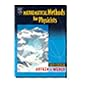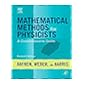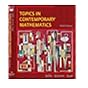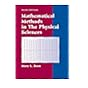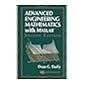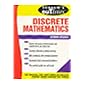510 ARM 2005 Mathematical methods for physicists / 510 ARM 2013 Mathematical methods for physicists : 510 BLT 2008 Topics in contemporary mathematics. 510 BOM 2006 Mathematical methods in the physical sciences / 510 DUA 2003 Advanced engineering mathematics with MATLAB / 511.0202 LIS 1976 Schaum's outline of theory and problems of discrete mathematics / 511.3130285 SII 2007 Introduction to fuzzy logic using MATLAB /

Includes biblographical references and index.

TOC Infinite series, power series --
Complex numbers --
Linear algebra --
Partial differentiation --
Multiple integrals --
Vector analysis --
Fourier series and transforms --
Ordinary differential equations --
Calculus of variations --
Tensor analysis --
Special functions --
Series solutions of differential equations; legendre, bessel, hermite, and laguerre functions --
Partial differential equations --
Functions of a complex variable --
Probability and statistics.

BA

Saifun Momota

There are no comments on this title.